## Search Here

### EQUATIONS FOR FINDING VOLUME

Cube = a3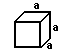Rectangular prism = a b c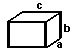Irregular prism = b h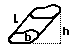Cylinder = b h =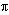r2 h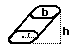Pyramid = (1/3) b h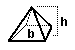Cone = (1/3) b h = 1/3r2 h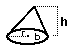Sphere = (4/3)r3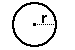Ellipsoid = (4/3) π r1 r2 r3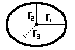<< View Formulas For Finding Area